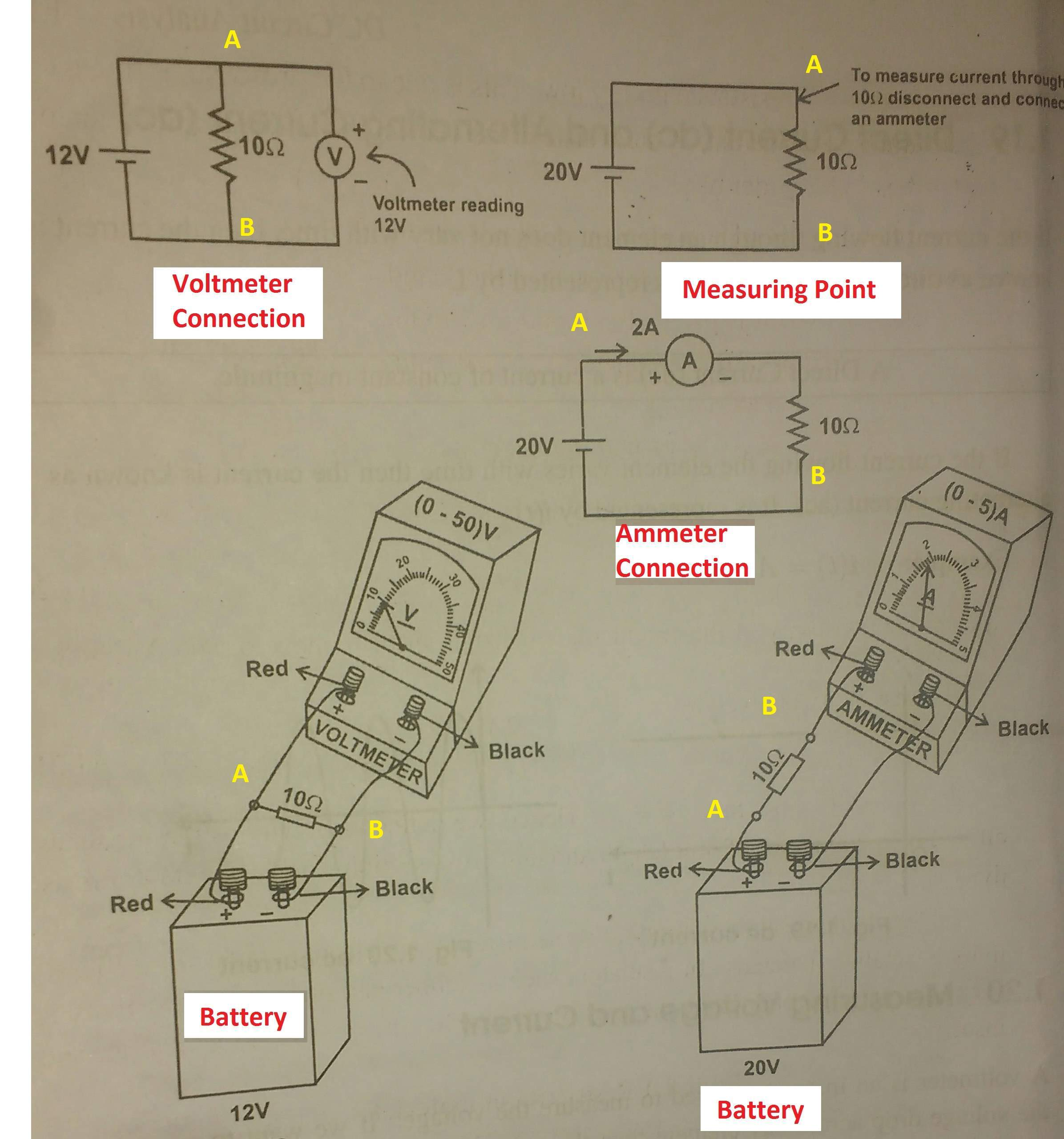# How to Measure Voltage and Current

## How to measure Voltage and current:?

Voltage and current is the important electrical quantity, To measure we need a special type of meter.. let see how to measure voltage and current in an electric circuit

## Measuring Voltage:

The voltage measuring instrument is called Voltmeter. If you want to measure a voltage across a component means, you should connect the voltmeter across the measuring components. The voltmeter is indicated by a small circle with a needle, which points out the digits.

Example: refer the diagram, I want to measure the voltage across the resistance R, then I have to connect the voltmeter across resistor, the point A and B. Voltage is an electrical potential or electrical pressure property, so to measure the voltage across any components, you do not want to disconnect the components from the circuit. The terminal polarity of the voltmeter and the component to be measured polarity should be same.

i.e Consider below diagram, If the point A is + ve terminal and point B is negative terminal means the voltmeter’s red terminal (Normally red consider as positive of the voltmeter) should be in Point A and black terminal (Normally black consider as negative terminal) should be in B. The Voltage will show in the voltmeter after completing all the arrangements.Note 1 Measuring Voltage: Before measuring the voltage, make sure the nature of voltage (AC Voltage or DC Voltage). We need different types of voltmeter, to measure different types of voltage…

Note 2 Measuring Voltage: Be aware measuring high voltage, to measure high voltage we need special equipment.

Note 3 Measuring Voltage: Make sure safety precaution.

Also using a voltmeter, we cannot measure the current flowing in the circuit.

## Measuring Current:

The Current measuring instrument is called ammeter. To measure the current flowing in a resistor or inductor or any components, the components should be disconnected and the ammeter should connect in series with that component. Because of current is flowing property (Flow of chares). To measure the current, you do not want to care about the polarity. Also using ammeter, we cannot measure Voltage in the circuit.

Key points for measuring current:

1. The ammeter should connect series with the load (to be measured)
2. Aware of maximum current can be flow through the circuit, to increase ammeter range for better result.